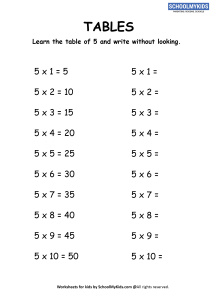# Math Worksheet : Multiplication Table 5

Learn and Practice Multiplication table of 5. Help you child learn multplication of 1 digit numbers using our multiplication tables and printable multiplicaiton table worksheets. Download and learn multiplication table 1-10 using this collection of multiplication tables 1-10 printable worksheets pdf.Viewed by 5885 parents and educators
Age Group